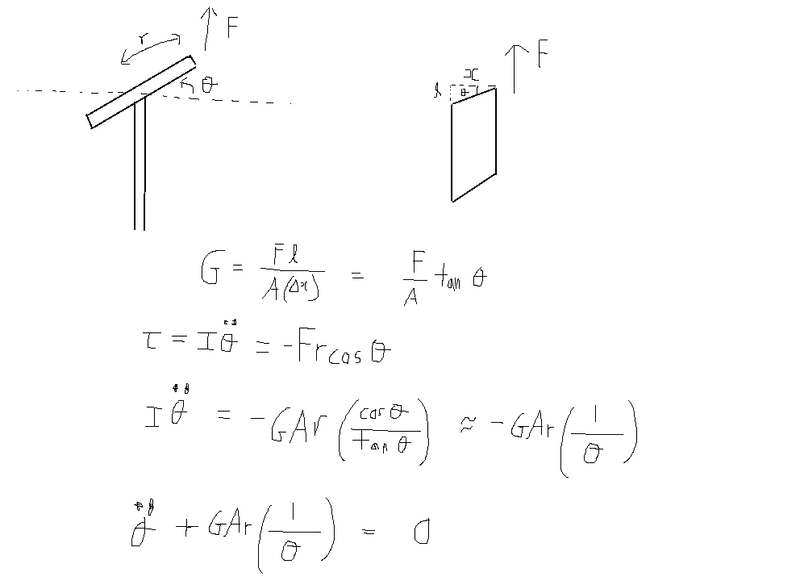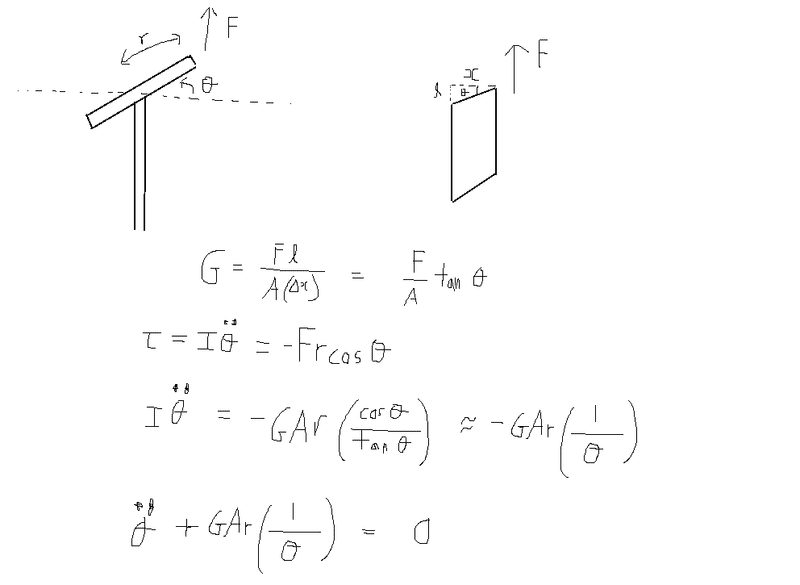# Small oscillation equation derivation

unscientific
Hi guys, I have been trying to find the "floppy" resonant mode frequency of a simple oscillator. The displacement is in the order of nanometers, while the dimensions of the oscillator is in cm. I think small angle approximations apply here. I got to the point of the equation of motion, but I have no idea how to solve it.

The equation for the shear modulus is defined. I used it to find the force.

I related force to torque.

I used maclaurin's expansion on cos θ and tan θ to approximate the equation in terms of θ.Would appreciate any help.

Last edited:

## Answers and Replies

Homework Helper
hi unscientific!you have θ'' = -C/θ

the standard trick to get a first integral is to multiply both sides by θ' to get …

θ'θ'' = -Cθ'/θ …

which integrates, and square-roots, to θ' = √(B - 2C*lnθ)(how you get past that, i've no idea)

•1 person
unscientific
Thanks tim, I've tried wolframalpha but it gave a non-analytical solution..

dauto
Expand C/θ in Taylor series around its equilibrium position and consider (by decree) that the higher order terms are negligible (That's what is usually meant by small oscillations)

dauto
No, wait, you can't do that. There seems to be something wrong with your equation. Expand cosθ around its equilibrium position instead.

dauto
Yes, there's definitely something wrong with your equation. The torque should be proportional to θ, not its inverse. Double check

unscientific
Yes, there's definitely something wrong with your equation. The torque should be proportional to θ, not its inverse. Double check

I can't see what's wrong with my equation..

dauto
You should have sinθ in your equation instead of cosθ. The angle θ in your picture is not the angle between F and r.

unscientific
You should have sinθ in your equation instead of cosθ. The angle θ in your picture is not the angle between F and r.

the torque is r x F, which gives cosθ instead.

nasu
Can you do a better (clearer) drawing? And what is the relationship between the two figures?

unscientific
Can you do a better (clearer) drawing? And what is the relationship between the two figures?

The figure on the right represents the part of the rod that's connected to the bob.

nasu
So that' part of a rod? I am sorry, I cannot imagine your system from these drawings.
Maybe someone else.

Is there a real system you are trying to model?

unscientific
So that' part of a rod? I am sorry, I cannot imagine your system from these drawings.
Maybe someone else.

Is there a real system you are trying to model?

A force is applied on the disc, which is attached to the rod. As a result, the rod flexes.

nasu
So what represent the right side of your drawing? The disk or the rod?

unscientific
So what represent the right side of your drawing? The disk or the rod?

the right side represents the flexing of the rod.

nasu
I am sorry. It's even more confusing for me. It looks like a sort of shear deformation to me.

unscientific
I am sorry. It's even more confusing for me. It looks like a sort of shear deformation to me.

Ok. The picture on the right is simply the part of the rod that is connected to the bob at the top. When the bob rotates, the rod flexes.

voko
I cannot see any picture at all.

unscientific
I cannot see any picture at all.

It's in the first post.

voko
It's in the first post.

It does not work for me. Can you attach it here or use some other image hosting facility?

unscientific
It does not work for me. Can you attach it here or use some other image hosting facility?

Does this work?voko
There are multiple problems with the derivation.

First, it is not correct to assume that the force is applied at some point and causes the rod to flex. Instead, the motion of the bob stresses the rod, and the restoring force (or torque) is generated. This torque is then applied to the bob, resulting in its oscillation.

Second, why are you dealing with the shear stress when the modes of vibrations you are after are flexural? My memory on the beam theory is rusty, but if I remember correctly, shear becomes important only with thick beams?

Third, can you really assume that all the flexing will be at the joint? It is much more reasonable to expect that the entire rod will be bending.